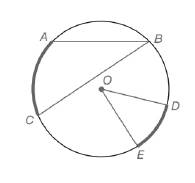Chapter 6.1, Problem 2E### Elementary Geometry for College St...

6th Edition
Daniel C. Alexander + 1 other
ISBN: 9781285195698

#### Solutions

Chapter
Section### Elementary Geometry for College St...

6th Edition
Daniel C. Alexander + 1 other
ISBN: 9781285195698
Textbook Problem
1 views

# For Exercises 1 to 8, use the figure provided.Exercises 1-8If m D E ⌢ = 46 ∘ ,  find m ∠ O .

To determine

To calculate:

The mO, by using the provided figure.

Explanation

Given:

The provided figure is,

Postulate used:

Central angle postulate:

In a circle, the degree measure of a central angle is equal to the degree measure of its intercepted arc.

Calculation:

To find: mO.

From the figure we see that O is the central angle.

Therefore, use central angle postulate.

From the figure, the intercepted arc is DE

### Still sussing out bartleby?

Check out a sample textbook solution.

See a sample solution

#### The Solution to Your Study Problems

Bartleby provides explanations to thousands of textbook problems written by our experts, many with advanced degrees!

Get Started

#### A sample of n = 9 scores has X = 108. What is the sample mean?

Essentials of Statistics for The Behavioral Sciences (MindTap Course List)

#### The unit vector in the direction of is:

Study Guide for Stewart's Multivariable Calculus, 8th

#### For f(x) = tanh1 2x, f(x) = a) 2(sech1 2x)2 b) 2(sech2 2x)1 c) 214x2 d) 114x2

Study Guide for Stewart's Single Variable Calculus: Early Transcendentals, 8th